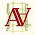## Saturday, May 6, 2017

### 1602

1.3.875 X 2.75 inches
Moulin du Roy 90 lb HP w/c paper
graphite/watercolor pencils/water-soluble wax pastels

the probability of a coin toss resulting in either 'heads' or 'tails' is 1
because there are no other options (assuming the coin lands flat)
the probability of .5 can be considered to have equal odds
of occurring or not occurring
the probability of a coin toss resulting in 'heads' is .5
because the toss is equally as likely to result in 'tails'
the probability of 0 can be considered an impossibility
the probability that the coin will land flat without either side facing up is 0 (landing flat either 'heads' or 'tails' will be facing up)

2.speaking of flat what is the probability of clement greenberg

1.formally... I'd say
a higher probability of inclement greenburg